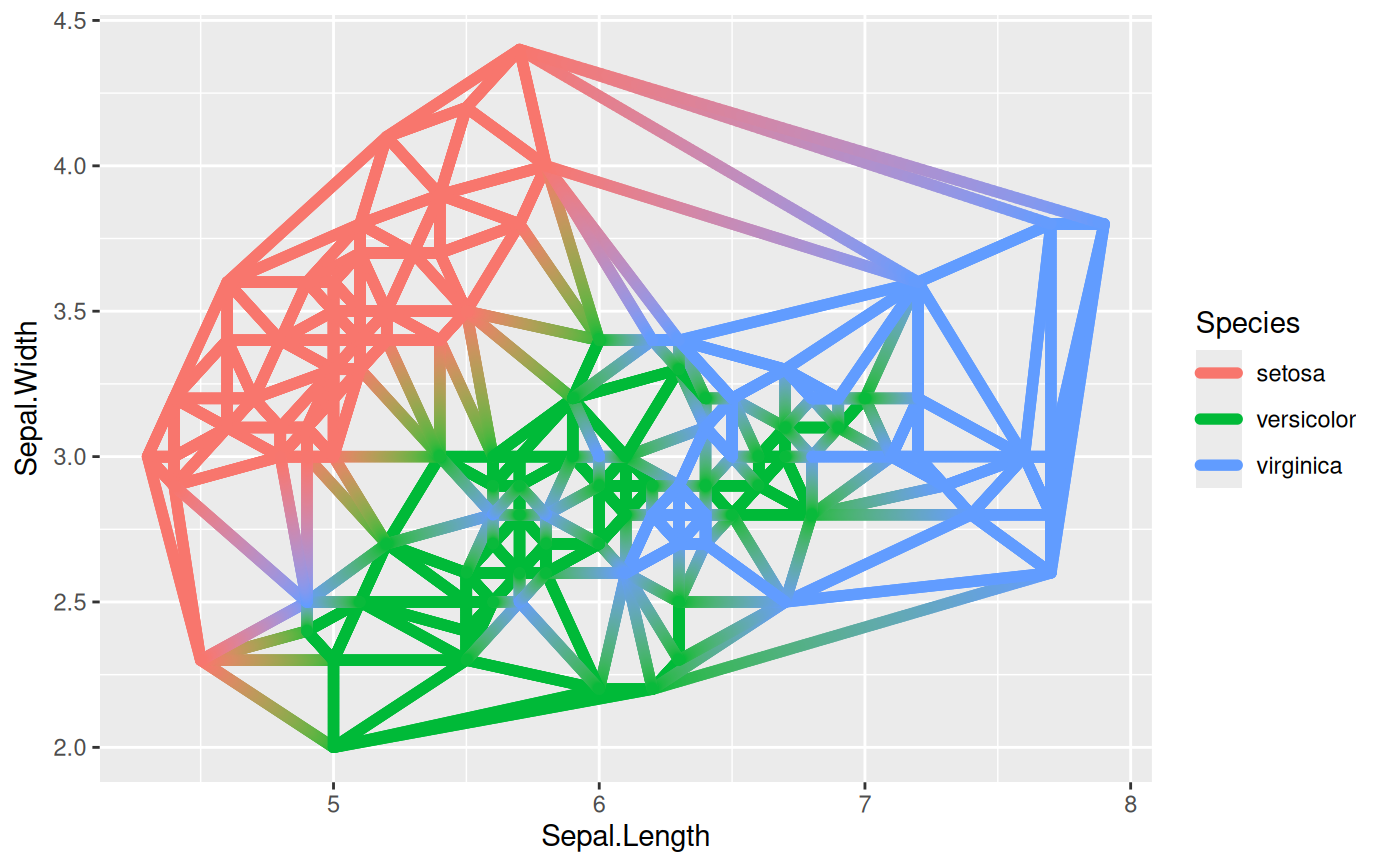This set of geoms and stats allows you to display voronoi tesselation and delaunay triangulation, both as polygons and as line segments. Furthermore it lets you augment your point data with related summary statistics. The computations are based on the deldir::deldir() package.

geom_voronoi_tile(
mapping = NULL,
data = NULL,
stat = "voronoi_tile",
position = "identity",
na.rm = FALSE,
bound = NULL,
eps = 1e-09,
normalize = FALSE,
asp.ratio = 1,
expand = 0,
show.legend = NA,
inherit.aes = TRUE,
...
)

geom_voronoi_segment(
mapping = NULL,
data = NULL,
stat = "voronoi_segment",
position = "identity",
na.rm = FALSE,
bound = NULL,
eps = 1e-09,
normalize = FALSE,
asp.ratio = 1,
show.legend = NA,
inherit.aes = TRUE,
...
)

geom_delaunay_tile(
mapping = NULL,
data = NULL,
stat = "delaunay_tile",
position = "identity",
na.rm = FALSE,
bound = NULL,
eps = 1e-09,
normalize = FALSE,
asp.ratio = 1,
expand = 0,
show.legend = NA,
inherit.aes = TRUE,
...
)

geom_delaunay_segment(
mapping = NULL,
data = NULL,
stat = "delaunay_segment",
position = "identity",
na.rm = FALSE,
bound = NULL,
eps = 1e-09,
normalize = FALSE,
asp.ratio = 1,
show.legend = NA,
inherit.aes = TRUE,
...
)

geom_delaunay_segment2(
mapping = NULL,
data = NULL,
stat = "delaunay_segment2",
position = "identity",
na.rm = FALSE,
bound = NULL,
eps = 1e-09,
normalize = FALSE,
asp.ratio = 1,
n = 100,
show.legend = NA,
inherit.aes = TRUE,
...
)

stat_delvor_summary(
mapping = NULL,
data = NULL,
geom = "point",
position = "identity",
na.rm = FALSE,
bound = NULL,
eps = 1e-09,
normalize = FALSE,
asp.ratio = 1,
show.legend = NA,
inherit.aes = TRUE,
...
)

## Arguments

mapping

Set of aesthetic mappings created by aes(). If specified and inherit.aes = TRUE (the default), it is combined with the default mapping at the top level of the plot. You must supply mapping if there is no plot mapping.

data

The data to be displayed in this layer. There are three options:

If NULL, the default, the data is inherited from the plot data as specified in the call to ggplot().

A data.frame, or other object, will override the plot data. All objects will be fortified to produce a data frame. See fortify() for which variables will be created.

A function will be called with a single argument, the plot data. The return value must be a data.frame, and will be used as the layer data. A function can be created from a formula (e.g. ~ head(.x, 10)).

stat

The statistical transformation to use on the data for this layer, either as a ggproto Geom subclass or as a string naming the stat stripped of the stat_ prefix (e.g. "count" rather than "stat_count")

position

Position adjustment, either as a string naming the adjustment (e.g. "jitter" to use position_jitter), or the result of a call to a position adjustment function. Use the latter if you need to change the settings of the adjustment.

na.rm

If FALSE, the default, missing values are removed with a warning. If TRUE, missing values are silently removed.

bound

The bounding rectangle for the tesselation or a custom polygon to clip the tesselation to. Defaults to NULL which creates a rectangle expanded 10\ vector giving the bounds in the following order: xmin, xmax, ymin, ymax. If supplied as a polygon it should either be a 2-column matrix or a data.frame containing an x and y column.

eps

A value of epsilon used in testing whether a quantity is zero, mainly in the context of whether points are collinear. If anomalous errors arise, it is possible that these may averted by adjusting the value of eps upward or downward.

The maximum distance a tile can extend from the point of origin. Will in effect clip each tile to a circle centered at the point with the given radius. If normalize = TRUE the radius will be given relative to the normalized values

normalize

Should coordinates be normalized prior to calculations. If x and y are in wildly different ranges it can lead to tesselation and triangulation that seems off when plotted without ggplot2::coord_fixed(). Normalization of coordinates solves this. The coordinates are transformed back after calculations.

asp.ratio

If normalize = TRUE the x values will be multiplied by this amount after normalization.

expand

A numeric or unit vector of length one, specifying the expansion amount. Negative values will result in contraction instead. If the value is given as a numeric it will be understood as a proportion of the plot area width.

As expand but specifying the corner radius.

show.legend

logical. Should this layer be included in the legends? NA, the default, includes if any aesthetics are mapped. FALSE never includes, and TRUE always includes. It can also be a named logical vector to finely select the aesthetics to display.

inherit.aes

If FALSE, overrides the default aesthetics, rather than combining with them. This is most useful for helper functions that define both data and aesthetics and shouldn't inherit behaviour from the default plot specification, e.g. borders().

...

Other arguments passed on to layer(). These are often aesthetics, used to set an aesthetic to a fixed value, like colour = "red" or size = 3. They may also be parameters to the paired geom/stat.

n

The number of points to create for each segment

geom

The geometric object to use to display the data, either as a ggproto Geom subclass or as a string naming the geom stripped of the geom_ prefix (e.g. "point" rather than "geom_point")

## Aesthetics

geom_voronoi_tile and geom_delaunay_tile understand the following aesthetics (required aesthetics are in bold):

• x

• y

• alpha

• color

• fill

• linetype

• size

geom_voronoi_segment, geom_delaunay_segment, and geom_delaunay_segment2 understand the following aesthetics (required aesthetics are in bold):

• x

• y

• alpha

• color

• linetype

• size

## Computed variables

stat_delvor_summary computes the following variables:

x, y

If switch.centroid = TRUE this will be the coordinates for the voronoi tile centroid, otherwise it is the original point

xcent, ycent

If switch.centroid = FALSE this will be the coordinates for the voronoi tile centroid, otherwise it will be NULL

xorig, yorig

If switch.centroid = TRUE this will be the coordinates for the original point, otherwise it will be NULL

ntri

Number of triangles emanating from the point

triarea

The total area of triangles emanating from the point divided by 3

triprop

triarea divided by the sum of the area of all triangles

nsides

Number of sides on the voronoi tile associated with the point

nedges

Number of sides of the associated voronoi tile that is part of the bounding box

vorarea

The area of the voronoi tile associated with the point

vorprop

vorarea divided by the sum of all voronoi tiles

## Examples

# Voronoi
# You usually wants all points to take part in the same tesselation so set
# the group aesthetic to a constant (-1L is just a convention)
ggplot(iris, aes(Sepal.Length, Sepal.Width, group = -1L)) +
geom_voronoi_tile(aes(fill = Species)) +
geom_voronoi_segment() +
geom_text(aes(label = after_stat(nsides), size = after_stat(vorarea)),
stat = 'delvor_summary', switch.centroid = TRUE
)
#> Warning: stat_voronoi_tile() is dropping duplicated points
#> Warning: stat_voronoi_segment() is dropping duplicated points
#> Warning: stat_delvor_summary() is dropping duplicated points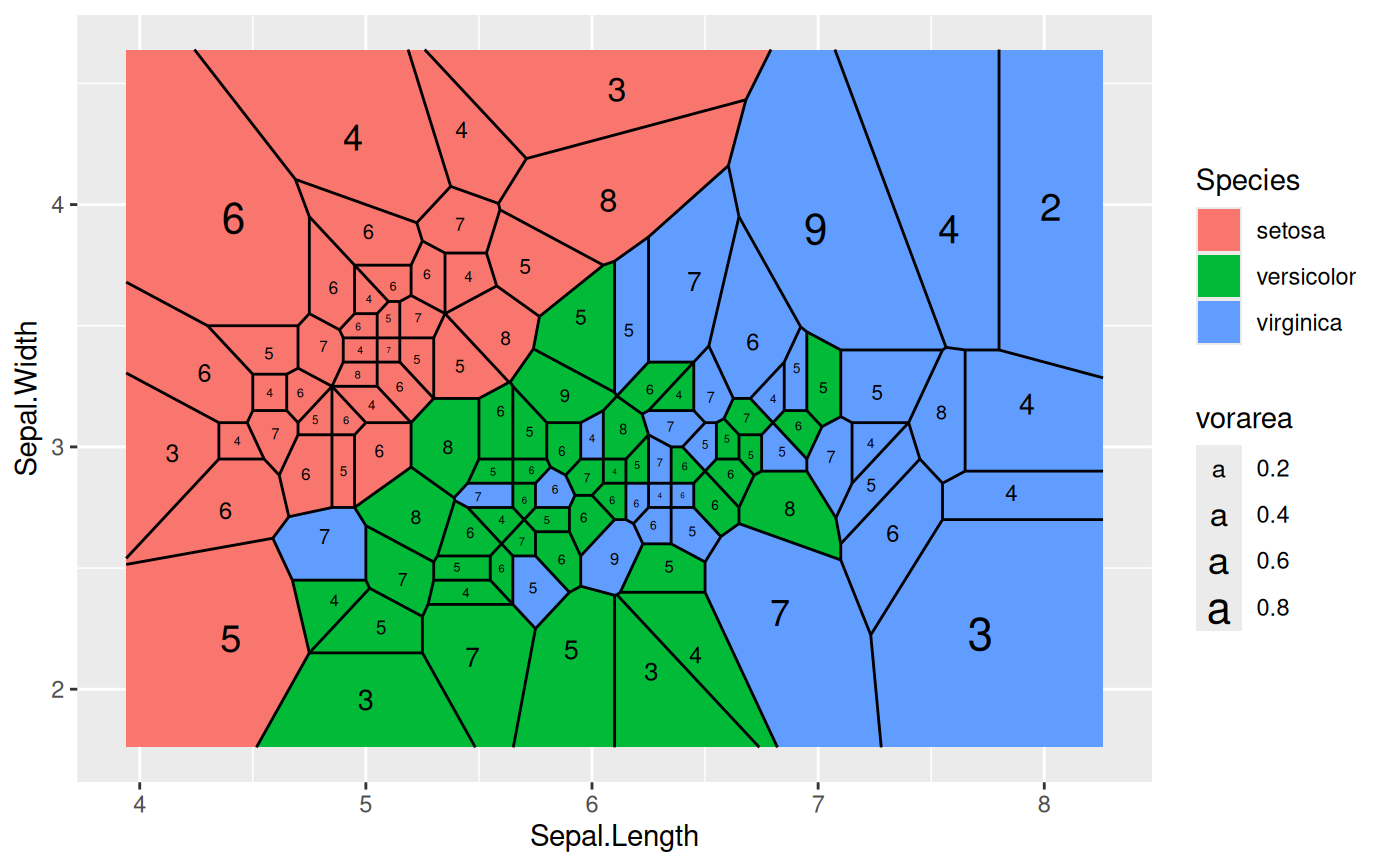# Difference of normalize = TRUE (segment layer is calculated without
# normalisation)
ggplot(iris, aes(Sepal.Length, Sepal.Width, group = -1L)) +
geom_voronoi_tile(aes(fill = Species), normalize = TRUE) +
geom_voronoi_segment()
#> Warning: stat_voronoi_tile() is dropping duplicated points
#> Warning: stat_voronoi_segment() is dropping duplicated points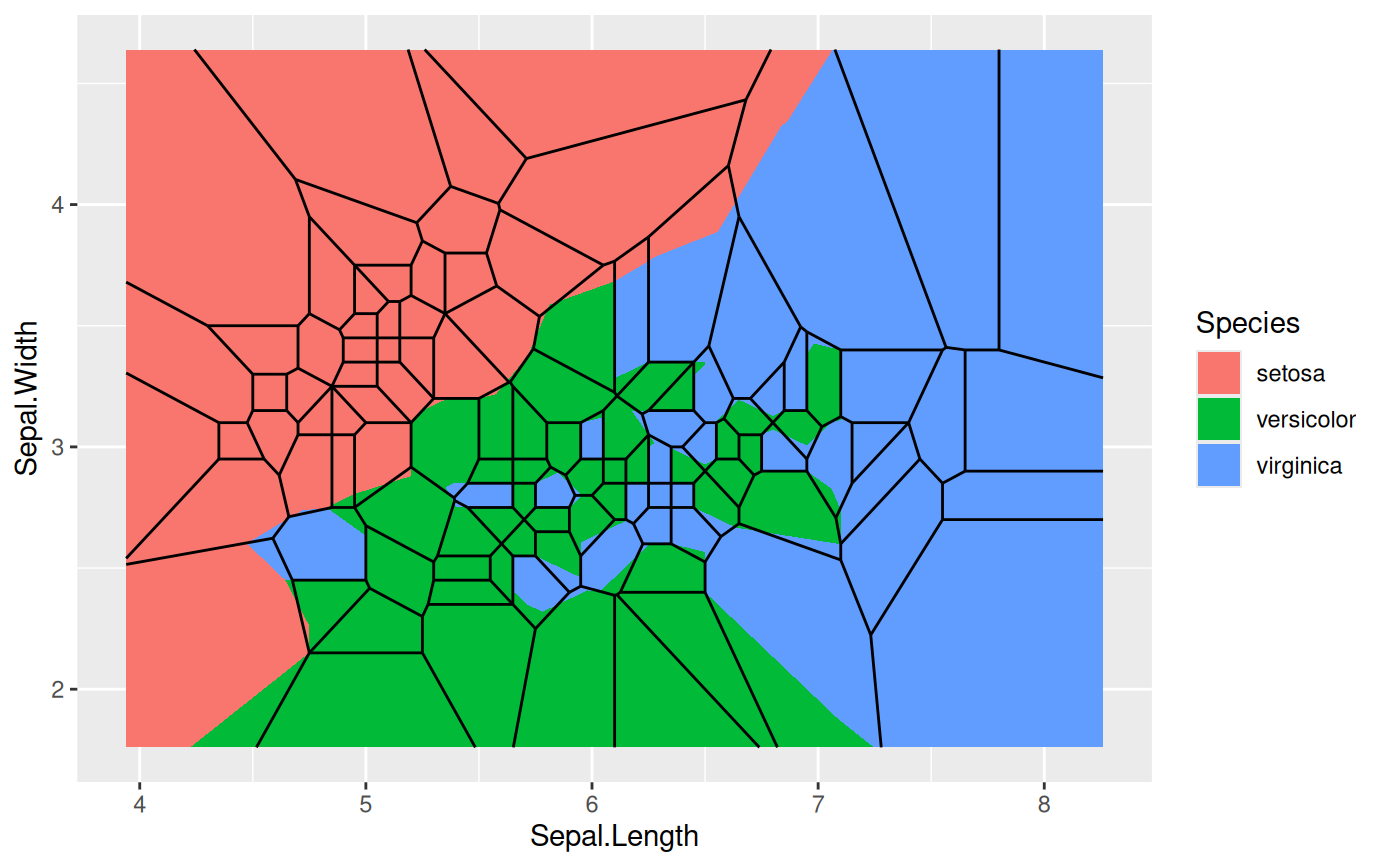ggplot(iris, aes(Sepal.Length, Sepal.Width, group = -1L)) +
geom_voronoi_tile(aes(fill = Species), colour = 'black', max.radius = 0.25)
#> Warning: stat_voronoi_tile() is dropping duplicated points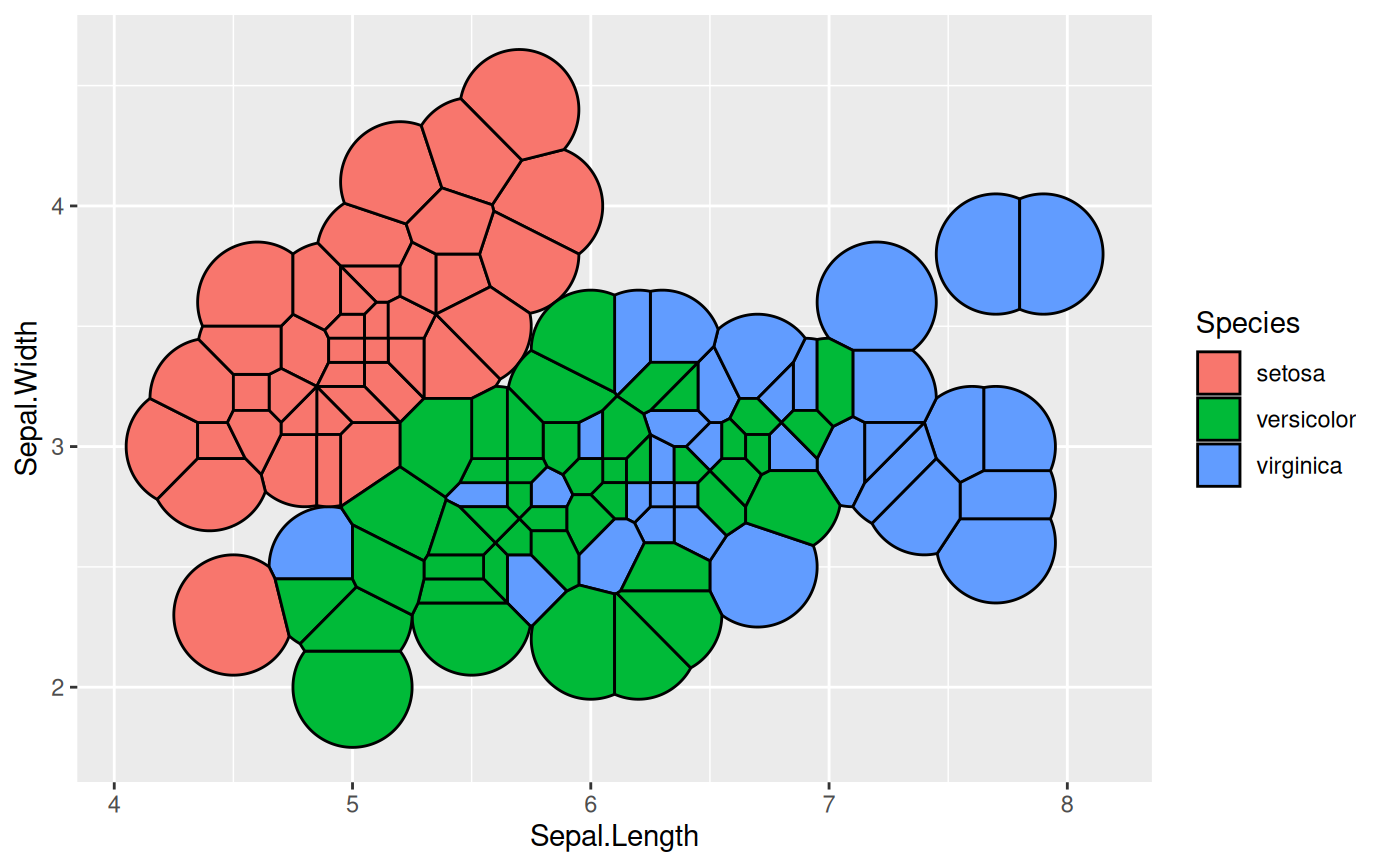# Set custom bounding polygon
triangle <- cbind(c(3, 9, 6), c(1, 1, 6))
ggplot(iris, aes(Sepal.Length, Sepal.Width, group = -1L)) +
geom_voronoi_tile(aes(fill = Species), colour = 'black', bound = triangle)
#> Warning: stat_voronoi_tile() is dropping duplicated points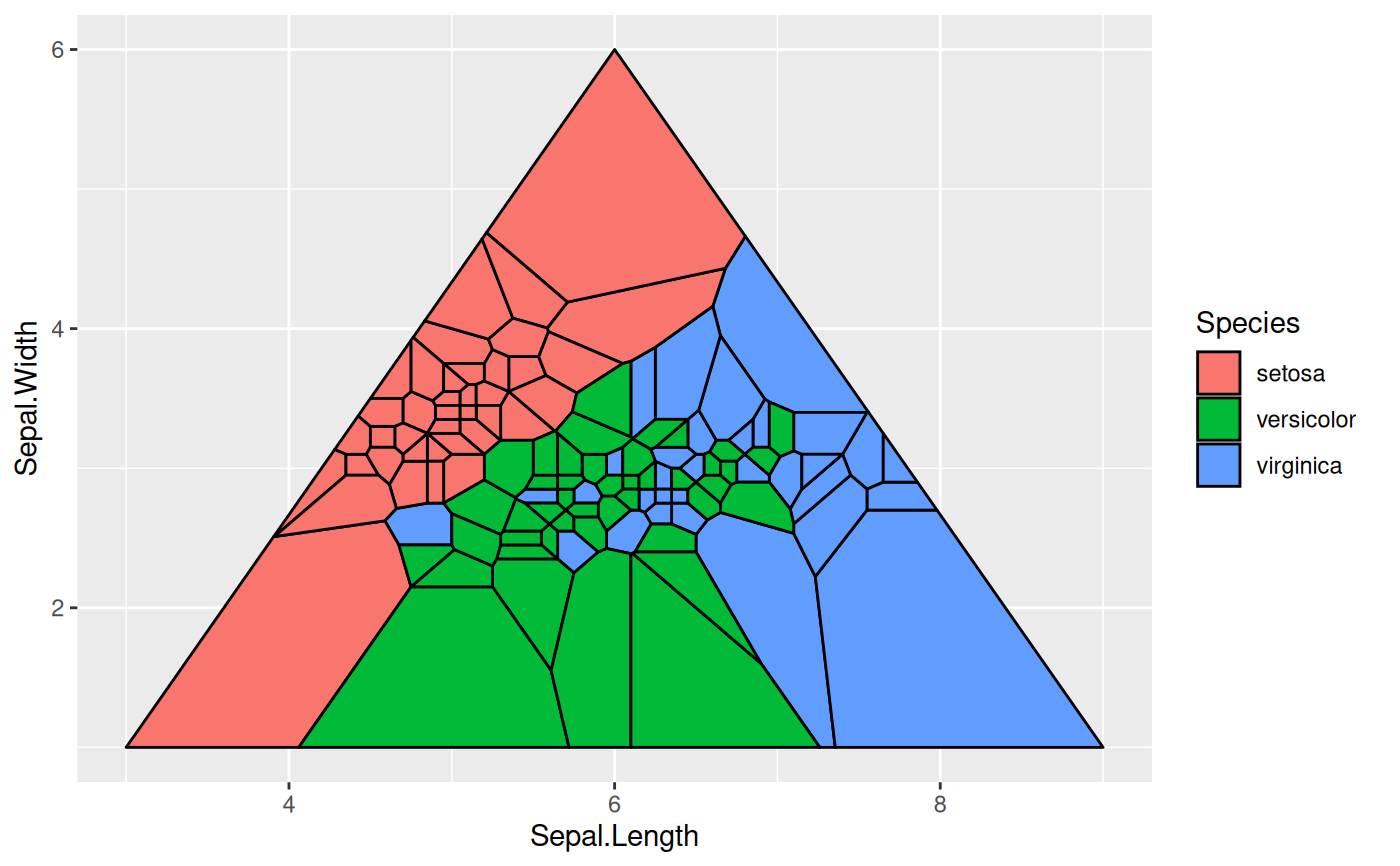# Use geom_shape functionality to round corners etc
ggplot(iris, aes(Sepal.Length, Sepal.Width, group = -1L)) +
geom_voronoi_tile(aes(fill = Species), colour = 'black',
expand = unit(-.5, 'mm'), radius = unit(2, 'mm'))
#> Warning: stat_voronoi_tile() is dropping duplicated points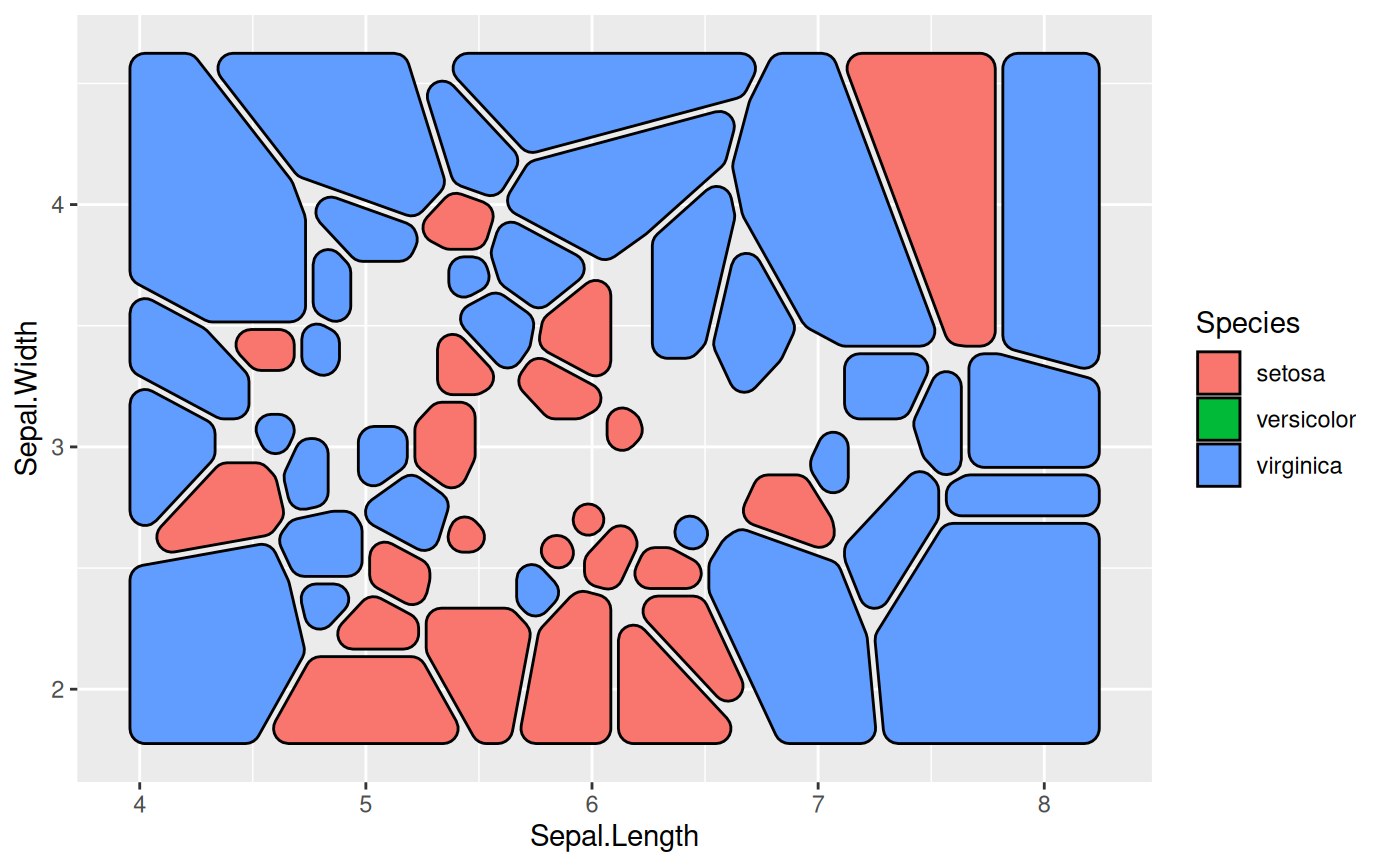# Delaunay triangles
ggplot(iris, aes(Sepal.Length, Sepal.Width)) +
geom_delaunay_tile(alpha = 0.3, colour = 'black')
#> Warning: stat_delaunay_tile() is dropping duplicated points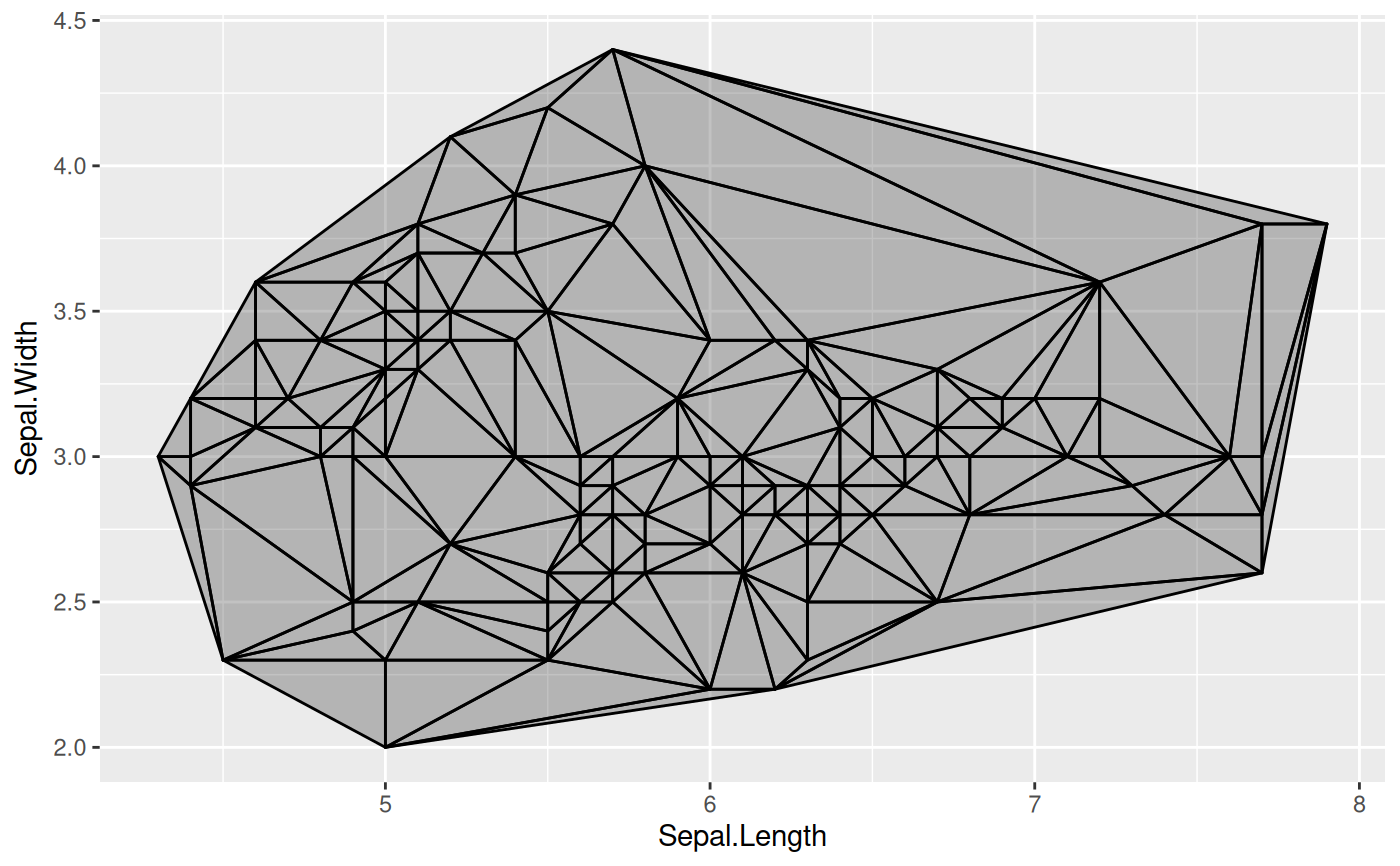# Use geom_delauney_segment2 to interpolate aestetics between end points
ggplot(iris, aes(Sepal.Length, Sepal.Width)) +
geom_delaunay_segment2(aes(colour = Species, group = -1), size = 2,
lineend = 'round')
#> Warning: stat_delaunay_segment2() is dropping duplicated points